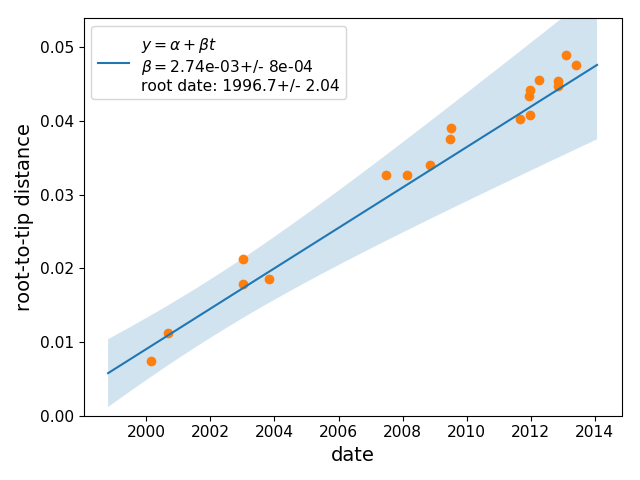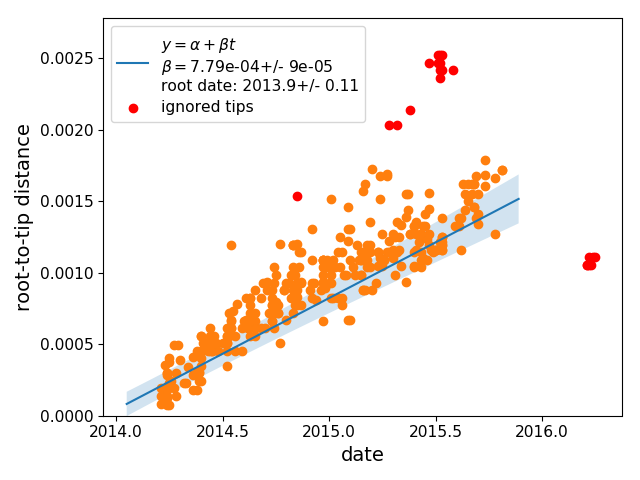# Estimation of evolutionary rates and tree rerooting

Treetime can estimate substitution rates and determine which rooting of the tree is most consistent with the sampling dates of the sequences. This functionality is implemented as subcommand `clock`:

```treetime clock --tree data/h3n2_na/h3n2_na_20.nwk --dates data/h3n2_na/h3n2_na_20.metadata.csv --sequence-len 1400 --outdir clock_results
```

This command will print the following output:

``` Root-Tip-Regression:
--rate:  2.826e-03
--r^2:   0.98

The R^2 value indicates the fraction of variation in
root-to-tip distance explained by the sampling times.
Higher values corresponds more clock-like behavior (max 1.0).

The rate is the slope of the best fit of the date to
the root-to-tip distance and provides an estimate of
the substitution rate. The rate needs to be positive!
Negative rates suggest an inappropriate root.

The estimated rate and tree correspond to a root date:

--- root-date:   1996.75

--- re-rooted tree written to
clock_results/rerooted.newick

--- wrote dates and root-to-tip distances to
clock_results/rtt.csv

--- root-to-tip plot saved to
clock_results/root_to_tip_regression.pdf
```

In addition, a number of files are saved in the directory specified with –outdir:

• a rerooted tree in newick format

• a table with the root-to-tip distances and the dates of all terminal nodes

• a graph showing the regression of root-to-tip distances vs time

• a text-file with the rate estimate## Confidence intervals of the clock rate

In its default setting, `treetime clock` the evolutionary rate and the Tmrca by simple least-squares regression. However, these root-to-tip distances are correlated due to shared ancestry no valid confidence intervals can be computed for this regression. This covariation can be efficiently accounted if the sequence data set is consistent with a simple strict molecular clock model, but can give misleading results when the molecular clock model is violated. This feature is hence off by default and can be switched on using the flag

```--covariation
```

## Filtering of tips

More often than not, a subset of sequences in an alignment are outliers and don’t follow the molecular clock model. Such outliers can badly skew rerooting and estimation of the substitution rates. To guard against such problems, `treetime clock` marks sequences as suspect if they deviate more than a certain amount from the clock model. TreeTime first performs a least-square root-to-tip vs date regression and then marks tips whose residuals are greater than `n` inter-quartile distances of the residual distribution. The parameter `n` is set via

```--clock-filter <n>
```

and is 3 by default. For the example Ebola virus data set, the command

```treetime clock --tree data/ebola/ebola.nwk --dates data/ebola/ebola.metadata.csv --sequence-len 19000
```